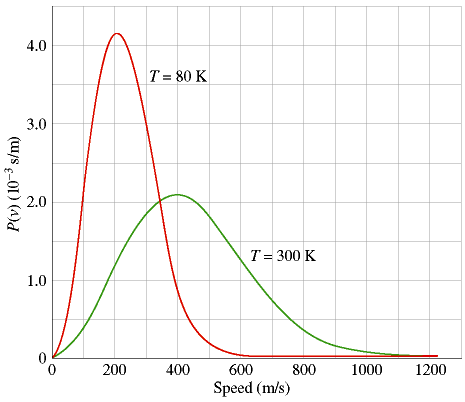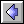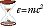### The Maxwell-Boltzmann DistributionThe Maxwell-Boltzmann Distribution is an equation, first derived by James Clerk Maxwell in 1859 and extended by Ludwig Boltzmann in 1868, which gives the probability that any given gas molecule in an ideal gas will be moving at a specific speed.  Even though we often talk of an ideal gas as having a "constant" temperature, it is obvious that every molecule cannot in fact have the same temperature.  This is because temperature is related to molecular speed, and putting 1020 gas molecules in a closed chamber and letting them randomly bang against each other is the best way I can think of to guarantee that they will not all be moving at the same speed.

The graph shows the Maxwell-Boltzmann speed-probability curve for an ideal gas at two temperatures.  Probability is plotted along the y-axis in more-or-less arbitrary units; the speed of the molecule is plotted along the x-axis in m/s.  This curve precisely connects the (instantaneous) speed of a gas molecule with the relative probability that it will have that speed.  Notice that the curves have prominent peaks, corresponding to the most probable speed of a molecule and to (approximately) the absolute temperature of the gas.  We should note two things:

1)  The Maxwell-Boltzmann curves are considerably different for a gas at 80 °K and at 300 °K.  When a gas becomes colder, not only does its peak shift to the left (lower temperature = slower molecules), but its peak also becomes higher and much more narrow.  At lower temperatures there is less energy available, so the gas molecules tend to have weaker collisions and to move at speeds that don't vary so much from each other.  In the limit where an ideal gas is taken to absolute zero, the molecules would stop moving altogether and therefore in fact be at constant speed.

2)  At higher temperatures, the Maxwell-Boltzmann curves flatten out.  There is more energy available, so now there are many more energetic collisions with which to potentially propel a molecule far faster than the average speed.  In particular, note the far right-hand region of both curves.  At 1000 m/s, the probability of a T=80 molecule having that speed is essentially zero. But for T=300 the probability is only small, not zero.  Therefore, even though the percentage of T=300 molecules moving at 1000 m/s is tiny by any standard, the ratio of the probabilities for the T=300 and T=80 gasses is quite large, because dividing by the T=80 case is almost like dividing by zero. In other words, relatively modest temperature increases in a gas can multiply the number of extremely hot, low-probability molecules by hundreds or thousands of times. This turns out to be critical for the energy production of stars, a topic I will discuss later in the course.Thermodynamics DatelineIdeas of Physics Homepage